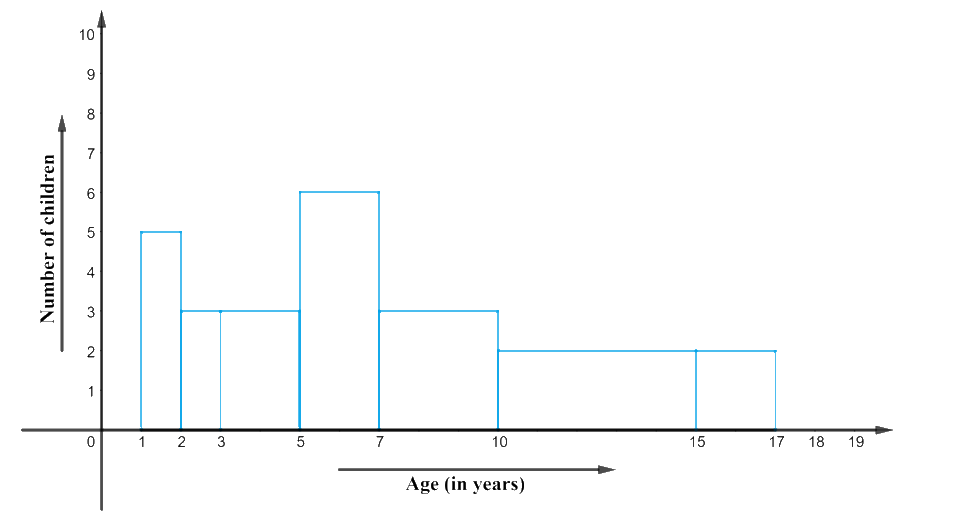# Ex.14.3 Q8 Statistics Solution - NCERT Maths Class 9

Go back to  'Ex.14.3'

## Question

A random survey of the number of children of various age groups playing in a park was found as follows:

 Age (in years) Number of children $$1 - 2$$ $$5$$ $$2 – 3$$ $$3$$ $$3 – 5$$ $$6$$ $$5 – 7$$ $$12$$ $$7 – 10$$ $$9$$ $$10 – 15$$ $$10$$ $$15 - 17$$ $$4$$

Draw a histogram to represent the data above.

Video Solution
Statistics
Ex exercise-14-3 | Question 8

## Text Solution

What is known?

A random survey of the number of children of various age groups playing in a park.

What is Unknown?

A Histogram to represent the data.

Reasoning:

(i) From the given data, we can observe that the class intervals have varying width. This will make the rectangular bars to have varying widths and will give us a misleading picture of the data.

(ii) The areas of the rectangles should be proportional to the frequencies in a histogram.

(iii) So we need to make certain modification in the lengths so that area’s again proportional to the frequencies. For that,

(a) Select a class interval in the minimum. Class size.

(b)The lengths of the rectangles are then modified to be proportionate to the class size $$1.$$

(c) For instance, when the class size is $$5,$$ the length of the rectangle is $$10.$$ So when the class size is $$1,$$ the length of the rectangle will be \begin{align}\frac{10}{5} \times 1=2\end{align}.

Steps:

We need to proceed in similar manner, to get the following table:

 Age (in years) Number of children Width of the Class Length of the rectangle $$1 - 2$$ $$5$$ $$1$$ \begin{align}\frac{5 \times 1}{1}=5\end{align} $$2 – 3$$ $$3$$ $$1$$ \begin{align}\frac{3 \times 1}{1}=3\end{align} $$3 – 5$$ $$6$$ $$2$$ \begin{align}\frac{6 \times 1}{1}=6\end{align} $$5 – 7$$ $$12$$ $$2$$ \begin{align}\frac{12 \times 1}{1}=6\end{align} $$7 – 10$$ $$9$$ $$3$$ \begin{align}\frac{12 \times 1}{1}=6\end{align} $$10 – 15$$ $$10$$ $$5$$ \begin{align}\frac{10 \times 1}{1}=2\end{align} $$15 - 17$$ $$4$$ $$2$$ \begin{align}\frac{4 \times 1}{1}=2\end{align}

Will take the age of children on $$x$$-axis and proportion of children per $$1$$ year interval per year on $$y$$-axis, the histogram can beLearn from the best math teachers and top your exams

• Live one on one classroom and doubt clearing
• Practice worksheets in and after class for conceptual clarity
• Personalized curriculum to keep up with school